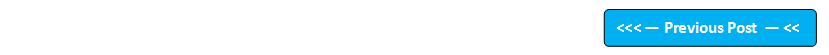Google+

## Blog Pages

### 57. 'do-while' iteration statementsAs we have seen, if the conditional expression controlling a while loop is initially false, then the body of the loop will not be executed at all. However, sometimes it is desirable to execute the body of a loop at least once, even if the conditional expression controlling the loop is  initially false.

do-while loop in Java always executes its body at least once, because it's conditional expression is at the bottom of the loop. Each iteration of the do-while loop first executes the body of the loop and then evaluates the conditional expression. If the condition is false, the loop terminates.

 Program to demonstrate that the do-while loop executes at least once even if the conditional expression controlling the do-while loop fails initially class DoWhile {   public static void main(String args[])   {      int i=6;       do       {             System.out.println("The value of i is "+i);       } while(i<6);  //Condition is at the bottom of the loop, hence the loop is executed at least once even if the condition initially fails    }  } Output of this program: The value of i is 6 do-while loop started without checking any condition and hence printed "The value of i is 6". After this it evaluated while(i<6) i.e. while(6<6) i.e. false, hence terminated the loop. This means even though the condition controlling the do-while loop initially fails, the statements inside the do-while loop will be executed one time. Another program to demonstrate the do-while loop class DoWhile2 {   public static void main(String args[])   {      int i=1;       do       {             System.out.println("The value of i is "+i);             i++;       } while(i<6);    }  } Output of this program: The value of i is 1 The value of i is 2 The value of i is 3 The value of i is 4 The value of i is 5 Program to demonstrate the infinite times executed  do-while loop class InfiniteDoWhile {   public static void main(String args[])   {      int i=1;       do       {             System.out.println("The value of i is "+i);       } while(i<6);    }  } Output of this program: 'The value of i is 1' will be printed infinite times In this program, we've not incremented the value of 'i' as we did in the previous program. Hence the do-while conditional expression always results in pass (i.e. 1<6) is always pass, hence it executes the statements inside the do-while block infinite times i.e. prints 'The value of i is 1' infinite times

Please comment below to feedback or ask questions.

How to use for loop will be explained in the next post.